# 1nvas0r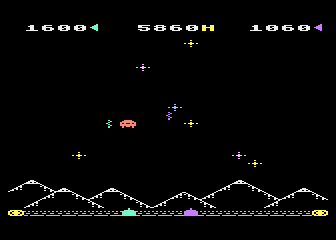This is a little game for the 2022 NOMAM's BASIC 10-liners Contest. This program fits in the EXTREM-256 category, and it was written using FastBasic 4.5.2 for the 8-bits ATARI XL/XE. Development started on 2021-09-12, and it took 3+1+2 days. The final version's date is 2022-02-22.

UPDATE: It obtained the 7th place of 17 entries in the category.

## Description

One invader, one button, one shoot, one life. Keep the Earth safe from a lot of single invaders.

## Instructions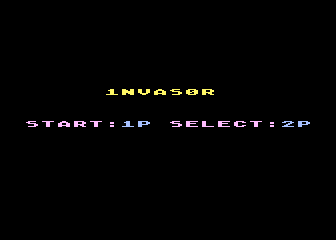When the title scren appears, press START to begin in single player mode, or SELECT for two players mode.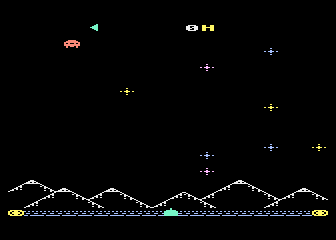In single player mode, you cannon moves from side to side, bouncing on the edges. An spaceship will appear from one side at the top. Each time it reaches the other side, it will descend one step and cross back to the other side. You can change the moving direction by shooting.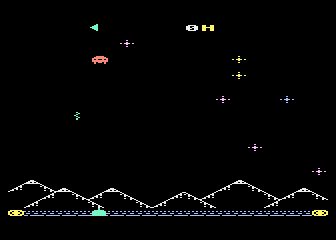Shoot with your cannon to destroy the ship. If you think your shot won't reach the invader, you can shoot again only if your bullet has already travelled a protion of the space. When you success, another ship will appear two steps higher.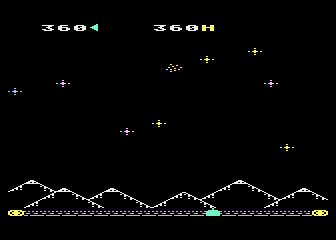Each time you hit and destroy an invader, your score will increase. The higher is the ship, the higher are the earned points. If you hit at the top, you will get a bonus.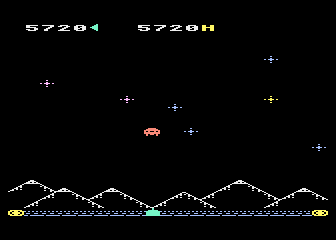When some amount of invaders have been destroyed, the following ones will go faster. After some waves of increasing speed, a slow wave will continue, but the number of invaders for the next increase will be less than the previous, and the delay for a repeated shooting will be increased.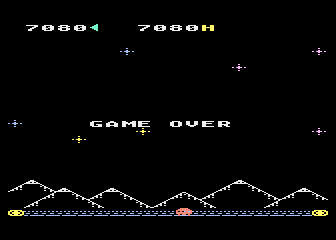If the invader reaches the earth and hits you, he won and the game is over. Press START key to protect the earth from another invasion. Press SELECT key to keep the earth safe with a companion.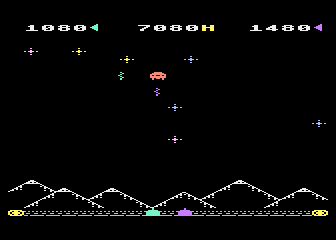In two players mode, both cannons share the same space, and they bounce between them.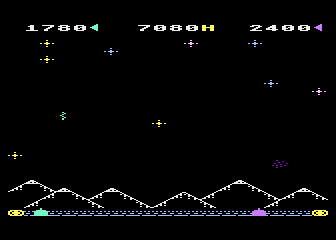Both can cooperate to avoid the invasion, or compete to see which one scores better.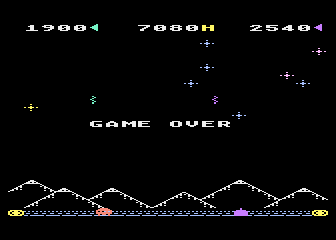The game is over when the spaceship reaches the earth and destroys any of the cannons. Press SELECT to start again in two players mode, or press START for a single player.

## Development of the game

I was connected live to the ZeroPage Homebrew show some months ago during the presentation of some new A8 homebrew games. One of the games was 1nvader, a 2021 port for the Atari XL/XE by Ken Jennings of a game for the C64 by Darren Foulds in 2019.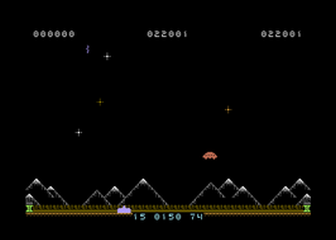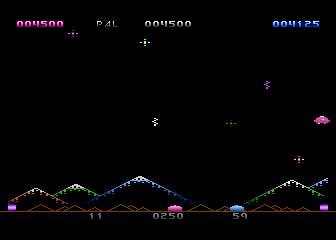During the show, interacting through the chat, I said that the game concept could be implemented as a BASIC tenliner, and some other people agreed, and joked about me releasing it in a couple of days, and that it could be released for 2022 contest. I took the challenge and wrote a prototype using FastBasic. After posting about this proto in Ken's thread at AtariAge and getting some replies, I decided to go for the full game. At that moment, the abbreviated source code for the proto had 5 lines for PUR-120 category of the contest. Extending the code to support two players using a different method to speed up things, the code increased to 12 lines.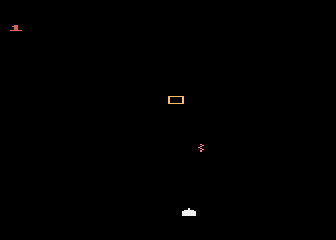Initial prototype.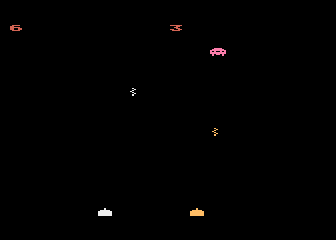Second prototype for two players.

As it was not possible to reduce the size of the source code, I decided to move it to the next category: EXTREM-256. That gave me almost 5 extra lines, where I could add code for the mountains and the starts. At the third day, the game was functional and very similar to the C64 version (not too colorful like the A8 one), but I added some gameplay improvements to make it more challenging, and it used only 8 lines of code. Then I forgot this project, waiting for the call for the contest.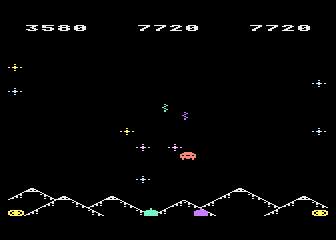Final prototype.

When the 2020 rules were published about 5 month later, it was time to check what was done. I didn't remembered a line of code. I opened the latest version in a text editor and found that only half of the code had comments. That was better than no comments at all. In order to add the remaining comments, I had to check and test what was each undocumented variable for. During this stage I found some bugs, which I could fix in a couple of minutes. I also did some fine tunning to the playfield, to make the game more enjoyable.

Main differences with the original 1nvader game are:

• There are only 4 waves of increasing speed instead of 8.
• Waves are of 10 spaceships before increasing the speed only for the first time. After every decrease of speed, waves are shorter by one spaceship.
• Except for the top row, score starts from 400 points and decreases by 20 points on every next row towards the bottom, instead of start at 500 and decrease by 25. The top row has a 1000 point reward in both games.
• You can't fire the laser until it reached a minimum of height if it has just been fired. In 1nvader the height is fixed, but in 1nvas0r it is moved a bit to the top every 4 waves.
• In 1nvas0r, the initial height margin is not the same for one player than two player modes, being higher for the later.
• The initial speed of the spaceship is higher in two players mode than in one player mode instead of being the same.
• Counters at the bottom of the screen were removed (they looked like debug info for me).

The final game, including a simple title screen, uses only 8 lines of FastBasic code. I could use the 2 remaining lines of code to improve the title screen, but I prefered to keep it simple.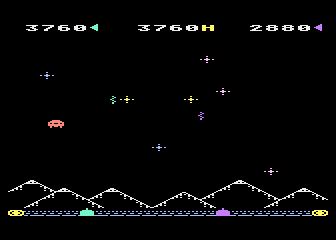Final game.

The name 1NVAS0R was selected because it is the Spanish word for "invader", but written with a "10" for tenliner.

Get the INVASOR.ATR file and set it as drive 1 in a real Atari (or emulator). Turn the computer on and the game should start after the loading completes. A joystick in port 1 is required.

NOTE: This game is for PAL computers. The ATR includes "NTSC.XEX" to be played on NTSC computers. I will run a bit faster than normal, but you will get the right colors.

## The code

The abbreviated BASIC code is the following: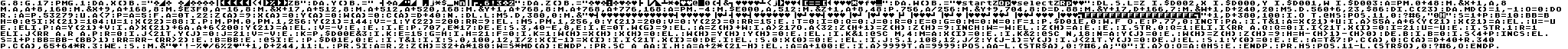The full and expanded BASIC listing is:

 1NVAS0R Tenliner port of 1NVADER (C64) (C) 2021-2022 Víctor Parada 2022-02-22 ```graphics 8 ``` Short way to clean 8K at the top of the RAM ```graphics 17 ``` Sets graphics mode 1 (ANTIC 6 mode) without text window ```pmgraphics 1 ``` Sets P/M graphics as single line resolution Data arrays for DLI. 23 bytes required for each one, as there are 23 interrupts. NOTE: Contained data is used for game initialization, then reused. This won't work in a ROM version of the game. ```data x() byte="{binary data}" ``` Invader position ```data y() byte="{binary data}" ``` Left cannon position ```data z() byte="{binary data}" ``` Right cannon position ```data w() byte="{binary data}" ``` Explosion position ```dli set l = z into \$D002, x into \$D000, y into \$D001, w into \$D003 ``` ```a=pmadr(0)+48 move adr(x)+9,a+168,8 ``` Sets up the cannon in P0 ```move adr(x)+1,a,8 move a,a+8,160 ``` Sets up the bullets into P0 ```move \$E3F0,a-16,8 ``` Sets the score pointer in P0 ```move adr(x)+17,a+512,8 move a+512,a+520,168 ``` Sets up the mothership in P2 ```move adr(y)+1,a+768,8 move a+768,a+776,168 ``` Sets up the explosion in P3 ```a=pmadr(-4) move \$E000,a,512 move adr(z)+1,a+8,48 poke 756,a/256 ``` Sets up the character set ```move adr(y)+9,704,8 ``` Sets the colors ```d=dpeek(88) move adr(y)+17,d+166,7 move adr(w)+1,d+240,20 ``` Prints the title screen ```mset dpeek(560)+6,23,\$86 ``` Enables DLI ```dim c(23) ``` Stores current position of the shining starts ```data md() = 1,-1 ``` Direction ```o=0 ``` High score ```do ``` Main loop ``` repeat a=peek(53279) until a<7 ``` Wait for a console key ``` p=a=5 ``` SELECT for 2 players (P=1), otherwise only 1 player (P=0) ``` for a=0 to 22 ``` Initializes P/M horizontal position arrays for DLI ``` z(a)=9 ``` Invader ``` x(a)=0 ``` Left cannon ``` y(a)=0 ``` Right cannon ``` w(a)=0 ``` Explosion ``` c(a)=d+40 next ``` Stars ``` dli l ``` Activates DLI ``` mset d,380,0 move adr("{binary data}")+1,d+380,100 ``` Set ups the screen, mountains with a clear sky ``` if o then exec hs position 11,0 print #6,"{binary data}" ``` Shows high score ``` s=1+p ``` Invader speed ``` b=10 ``` Current invaders count for current round ``` bb=b ``` Invaders max count for the next round ``` h=0 ``` Invader height ``` exec si ``` Invader direction ``` x(21)=104 u=1 ``` Left cannon setup ``` x(22)=88 ``` Left score pointer (P/M horizontal position wraps from the bottom of the screen) ``` if p ``` 2nd player? ``` move pmadr(0),pmadr(1),256 ``` Right cannon setup ``` y(21)=144 ``` Sets its position at the right of the left cannon ``` v=-1 ``` It starts moving to the left ``` y(22)=200 ``` Enables right score pointer ``` rr=9 ``` Min height of previous shot for a new shooting is higher than in one player mode ``` else ``` No second player, so ``` mset pmadr(1),256,0 ``` No right cannon ``` y(21)=200 ``` Sets the limits for the left cannon at the right side ``` v=0 ``` Right cannon does not move ``` y(22)=0 ``` No right score pointer ``` rr=15 endif ``` Min height of previous shot for a new shooting is lower than in two players mode ``` t=0 ``` Timer ``` i=0 q=0 j=0 r=0 ``` Shoot ``` e=0 g=0 ``` Explosion ``` m=0 n=0 ``` Score ``` f=1 ``` Game over flag ``` poke \$D01E,0 ``` Clear collisions ``` while f or e ``` Game loop Repeat until one of the players is dead (and the end of the last explosion) ``` inc t pause ``` ``` if t&1 ``` Moves the cannons, only one on each iteration ``` a=x(21)+u if a>55 and a+6x(21)+6 and a+6<200 ``` Checks if the next position of the right cannon is within the left cannon position and the right border ``` y(21)=a else ``` Updates right cannon position ``` v=-v endif endif ``` Changes right cannon direction ``` if e ``` Explosion? ``` poke 707,e*17 ``` Modifies explosion color, every time a darker one ``` dec e ``` Updates the explosion timer ``` sound 3,40-e,8,e ``` Explosion sound ``` if e=0 then w(g)=9 ``` Removes the explosion image when animation is complete ``` else a=z(h)+w ``` Move the ship ``` if a>217 or a<32 ``` Out of bounds? ``` z(h+1)=z(h) z(h)=9 inc h ``` Move to next row ``` w=-w ``` Changes direction ``` else z(h)=a endif endif ``` Moves horizontally ``` a=t&7 ``` Twinkle stars ``` poke c(a),0 ``` Removes a star from its previous position ``` c(a)=d+40+rand(340) ``` Chooses a new position for the star ``` poke c(a),65+64*rand(3) ``` Displays a star in a random color ``` if strig(0) ``` Enables shoot for left player ``` q=1 ``` Allows next shoot when the trigger is released ``` elif i1)-(h>0) ``` Sets the new height of the ship two layers far (except in the top ones) ``` dec b ``` Decreases the number of remainin ships for the round ``` if b=0 ``` End of round? ``` if s<4+p ``` Checks for maximum speed ``` inc s else ``` Not yet, increases speed ``` s=1+p ``` Slows down ``` bb=bb-(bb>1) ``` Decreases the number of targets for the next round ``` rr=rr-(rr>2) endif ``` Raises the height of current bullet for a new shoot ``` b=bb endif ``` Sets remaining ships for next round ``` exec si endif ``` Sets up the new invader ``` poke \$D01E,0 endif ``` Clear collisions ``` poke 77,0 ``` Disables attract mode ``` if t&1 ``` Move one bullet only on every iteration ``` if i sound 0,100,12,i/2 ``` Move left bullet ``` x(i-1)=x(i) ``` Copies the bullet horizontal position from the previous height or from its cannon ``` if i<21 then x(i)=0 ``` Removes the bullet if it was already on screen ``` dec i else ``` Updates bullet height ``` sound 0 x(0)=0 endif else ``` No left bullet ``` if j sound 1,108,12,j/2 ``` Move right bullet ``` y(j-1)=y(j) ``` Copies the bullet horizontal position from the previous height or from its cannon ``` if j<21 then y(j)=0 ``` Removes the bullet if it was already on screen ``` dec j else ``` Updates bullet height ``` sound 1 y(0)=0 endif endif ``` No right bullet ``` wend ``` ``` sound ``` Shuts off pending sounds ``` move adr("{binary data}")+1,d+244,11 ``` Game Over message ```loop ``` ```proc si ``` Set invader ``` a=rand(2) ``` Chooses side ``` z(h)=32+a*180 ``` Puts the invader at the chosen side ``` w=s*md(a) endproc ``` Assigns the moving direction according to the side ```proc sc a aa ``` Updates a score, returns new score in A ``` if h ``` Where was the ship? ``` a=a+2*(21-h) else ``` Score depends on the height of the ship ``` a=a+100 endif ``` Top row has a bonus ``` if a>9999 then a=9999 ``` Limits the score to 99990 ``` position aa-len(str\$(a)),0 print #6,a;"0" ``` Displays the score for the current player ``` if a>o o=a exec hs endif endproc ``` New record? ```proc hs position 11-len(str\$(o)),0 print #6,o endproc ``` Displays high score (without trailing "0H")